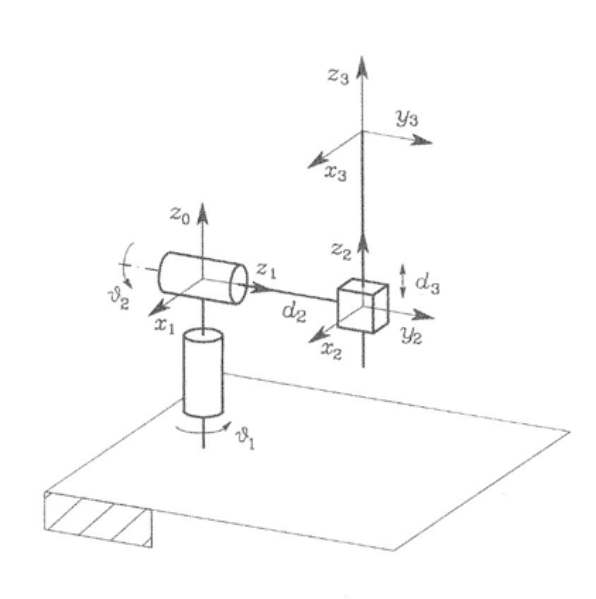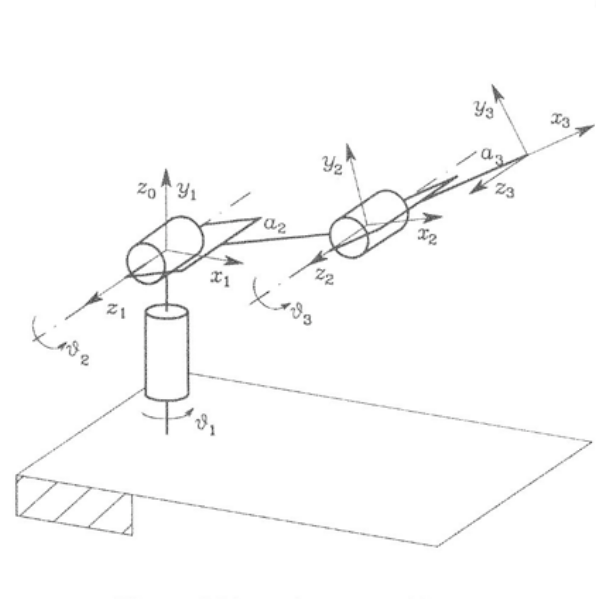# Forward Direct KinematicsIn this series of tutorials, we’ll go over the basics of manipulator robot kinematics. This involves parameterizing the geometry of the robot in a standardized fashion, allowing us to compute the pose of each link - including the endeffector, where the robot typically interacts with the environment - in terms of the joint variables. This is known as direct kinematics. We’ll then present differential kinematics which defines how changes in the joint variables relate to changes in link poses. Finally, we’ll investigate the problem of inverting the kinematics equations in order to compute how the joints need to be controlled to achieve a desired motion of the robot’s endeffector.

# The Fixed-Base Manipulator

Let’s begin by introducing the type of robot we’ll initially be modeling and controlling in our simulator - a fixed-base manpulator.

The robot consists of a chain of rigid links connected by controllable joints - typically electric motors or pneumatic/hydraulic piston linkages. One end of the chain is constrained to a base - for example, rigidly fixed to the ground or a tabletop - while the other end is connected to an endeffector - for example, a gripper - for interaction with the environment.Fixed-base manipulators are a good place to start learning about kinematics, dynamics and control; much of the theory translates to legged robots, with the main difference being that manipulators are inherently stable (can’t fall over).

Consider a simple grasping task shown below; the three key components of manipulator control we’ll go over are manipulator forward kinematics, endeffector path planning and manipulator inverse kinematics.These form a sort-of closed loop control - starting from forward kinematics to determine where the gripper is in space, followed by path planning and inverse kinematics for manipulator trajectory planning, and then joint-level control to move individual joints according to the plan.

In this post we’ll start with forward kinematics, learning how to parameterize the manipulator geometry based on a standardized form so that we can compute the pose of each link from measured joint angles.

# Forward (Direct) Kinematics

The goal of forward (direct) kinematics is to find a mapping - via a sequence of homogeneous transformations - which descibes the pose of the end-effector with respect to the base in terms of the joint variables.

## A note on terminology

The term forward in this context refers to a function which takes the joint state as input and outputs the Cartesian state of link(s). More generally, we say that forward kinematics maps from the joint space to the so-called operational space where we “operate on” (interact with) the environment. As we’ll introduce later, the opposite is inverse kinematics which maps from the operational space back into the joint space.

The term direct refers to the fact that we’re mapping from joint positions to operational space Cartesian pose. It’s also useful to work with differential kinematics, which maps joint velocities to Cartesian twist (linear + angular velocity).

So, in total, we have four different ways of looking at manipulator kinematics - forward direct, forward differential, inverse direct, and inverse differential. We’ll talk about forward direct kinematics in this post and forward differential kinematics in the next post. We’ll leave all of inverse kinematics to a future post.

## Problem setup

Consider an open chain of $n+1$ links connected by $n$ joints as shown below.Here, the first link - Link 0 - is the fixed base. Each joint, denoted by $q_{i}$, provides the structure with a single degree of freedom (DOF). Notice that joint $q_{i-1}$ is always the first to affect link $i$; this difference in indexing is a common cause of confusion.

Attached to each link from 0 (base) to $n$ is the link frame denoted by $L_{i}$; the coordinate transformation from frame $n$ to frame $0$ is then

where each homogeneous transformation $A_{i}^{i-1}$ is incremental (defined relative to the preceding link) and is thus a function of only one joint variable.The direct kinematics function from the end-effector to the base also requires transformations from link 0 to the base and from link $n$ to the end-effector; these are typically constant transformations. The direct kinematics function is thus written as

where we introduce $\mathbf{q}=[q_{0},q_{1},\ldots,q_{n-1}]$ to denote the vector of all joint states.

In the previous section, we described loosely how direct kinematics allows us to compute the homogeneous transform of the endeffector - or any frame in between - relative to the base. We also introduced link frames and made the choice to locate them each at the origin of the preceding joint. However, we didn’t actually describe how to define the axes of each link frame - and as it turns out, there are a number of reasonable ways to go about this. Probably the most well-known is the Denavit-Hartenberg convention.

### Denavit-Hartenberg Convention

This convention describes how to define the link frames such that computation of the direct kinematics function is accomplished in a general, systematic fashion. It’s a bit complicated to visualize in three dimensions, so if it’s still confusing I suggest checking out this video which does a great job at visualizing the process of defining frames. That said, the basic rules are as follows.

The frame corresponding to the $i^{th}$ link is located at joint $i-1$ and is defined as follows:

• Choose the z-axis $z_{i}$ of each frame through the axis of the joint.
• Locate the origin of the frame at the intersection of $z_{i}$ and the common normal to $z_{i}$ and $z_{i-1}$. If $z_{i}$ and $z_{i-1}$ intersect, this is the location of the origin.
• Choose the x-axis $x_{i}$ along the common normal to $z_{i}$ and $z_{i-1}$. If these z-axes intersect, choose $x_{i}$ to be $z_{i-1}\times z_{i}$.
• Finally, choose $y_{i}$ to complete a right-handed coordinate frame.

Consider the three-link planar (z-axes are out of the page) manipulator having three revolute joints as below:1 θ1 0 0 a1
2 θ2 0 0 a2
3 θ3 0 0 a3

In total, there are $n$ links and $n+1$ frames (one at each joint and one at the endeffector). With the frames chosen as above, we can uniquely specify the manipulator geometry with four parameters:

• $\theta_{i}$ is the angle between $x_{i}$ and $x_{i-1}$ about $z_{i-1}$. It’s only variable for a revolute joint, otherwise it’s a fixed offset angle.
• $d_{i}$ is the distance between the origins of frames $i$ and $i-1$ along the direction of axis $z_{i-1}$. It’s only variable for a prismatic joint, otherwise it’s a fixed distance offset. Obviously $d_{i}=0$ for a planar manipulator like the one above.
• $\alpha_{i}$ is the angle between axes $z_{i-1}$ and $z_{i}$ about $x_{i}$; this is always a fixed offset angle.
• $a_{i}$ is the distance between the axes $z_{i-1}$ and $z_{i}$ along the direction of axis $x_{i}$ (common normal between z-axes); this is always a fixed offset distance.

Note that we consider each joint to have one DOF, meaning only one of $\theta_{i}$ and $d_{i}$ can be variable for each link. More complex joints having multiple DOFs (eg spherical joints like your shoulder) are treated as combinations of single-DOF joints sharing the same origin. More on that later.

In this setup, Link $i$ is located between Frames $i$ and $i-1$; the homogeneous transformation between these frames as a general function of the DH parameters $\{\theta,d,\alpha,a\}$ is given by

Consider again the three-link planar arm, for which we’ve highlighted the homogeneous transformation between the first and second link:Note that since $\alpha_{2}=0$ and $d_{2}=0$, the rotational and translational portions of the transformation matrix $A_{2}^{1}$ are two-dimensional, as expected for a planar arm. This is not the case for more complex manipulators, as we will see below.

The ultimate goal is generally to compute the endeffector pose (rotation + translation), since this is usually the point on the robot which interacts with the world. Since the three links are identical in this case, the homogeneous transformation between successive links is

where we introduce $\cos{x}=c_{x}$ and $\sin{x}=s_{x}$ for brevity. The homogeneous transformation which describes the pose of the endeffector relative to the base is composed as intriduced earlier:

Substituting in the transform definitions (including the base to frame zero transform, which is the identity since they are identical frames) we have

which multiplies out to

where we introduce the notation $c_{xy}=\cos{x}\cos{y}$ and $s_{xy}=\sin{x}\sin{y}$ and so forth. Illustrated graphically:Whew! Let’s check out some more complex manipulator structures now. Disclaimer: I copied the following diagrams from the aforementioned text (Siciliano et al) but plan to revisit this and create some nicer-looking 3d diagrams for these structures.

#### Case study 2: spherical arm

The spherical arm consists of two co-located revolute joints, followed by our first prismatic (translation) joint. Note that both $\theta_{2}$ and $d_{2}$ are nonzero; however, only one of these can be variable for a single joint. In this case, $\theta_{2}$ is the joint’s DOF and $d_{2}$ is fixed. This is made clear from the use of a cylinder to illustrate the joint; a prismatic joint is shown as a cube, eg for the DOF $d_{3}$.1 θ1 0 -π/2 0
2 θ2 d2 π/2 0
3 0 d3 0 0

#### Case study 3: anthropomorphic arm

The anthropomorphic arm is essentially a vertical two-link planar arm (latter two joints) with the first DOF providing rotation of the remainder of the structure within the (ground) plane.